## Tuesday, May 2, 2017

### On Thermal Expansion & Thermal Contraction - 18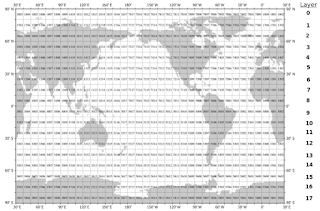Fig. 1  World Ocean Database layers
I. Background

The major cause of sea level change, at the most basic level, is global warming induced climate change.Fig. 2 Layer Zero
The next level after that is the dynamics that change the mass and/or volume of the oceans.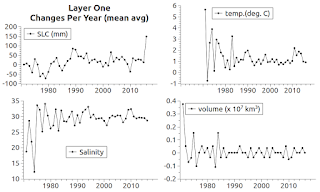Fig. 3 Layer One
It is important to know what these dynamics are, because the security of civilization as we know it is at stake (Climate Central).Fig. 4 Layer Two
In the previous post I presented graphs that compared ocean water temperature and salinity change to sea level change (SLC).Fig. 5 Layer Three
In today's graphs I have added another factor, steric volume change.Fig. 6 Layer Four
The ocean areas in today's post are identical to those in the previous post.

That is, both feature Layer Zero - Sixteen (compare Fig. 1 with Fig. 1 On Thermal Expansion & Thermal Contraction - 17).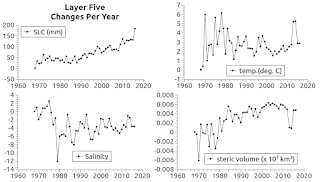Fig. 7 Layer Five
The fourth pane (lower right pane), in each graph today, depicts the change in volume of each layer of the ocean.Fig. 8 Layer Six
Those panes and the data are totally unrelated to the PSMSL data utilized to make the SLC graph in pane one (upper left pane) of each graph.Fig. 9 Layer Seven
I mention that because generating the data in pane four is orders of magnitude more difficult than any of the other panes in each graph.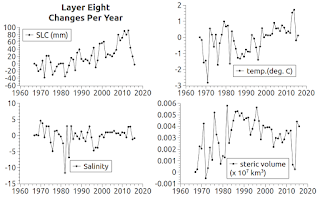Fig. 10 Layer Eight

II. Let Me Explain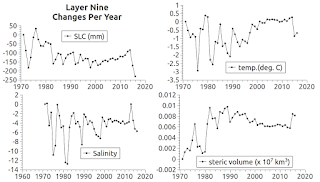Fig. 11 Layer Nine
Layer Nine First, let's consider the difficulty of calculating the volume of a layer.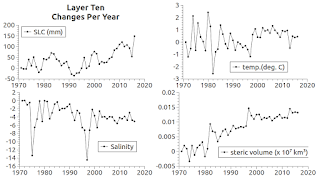Fig. 12 Layer Ten
When one views the map at Fig. 1, at first blush it looks like a simple volume formula for a square will do (v = l * w * h).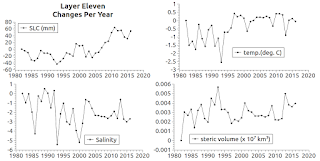Fig. 13 Layer Eleven
But the equal-sided looking latitude, longitude "squares" are not squares because they are on a globe, a sphere.Fig. 14 Layer Twelve
The four sides are no longer equal, nor flat anymore (since librul scientists changed the flat earth into a globe: Once Upon A Time In The West - 2).Fig. 15 Layer Thirteen
So, to determine the lengths of each side of each zone, one must calculate using some trigonometric formulas.

This involves sine, cosine, tangent, etc. values relating to the latitude and longitude lines that make up the four sides of each zone.Fig. 16 Layer Fourteen
An interesting aside is that this also means is that the seawater volume of the zones touching the Equator is greater than the seawater volume of zones nearer to polar regions.Fig. 17 Layer Fifteen
That is, the least seawater volume per zone is in Layer Zero and Layer Seventeen, while the most seawater per zone is in Layer Eight and Nine.Fig. 18 Layer Sixteen
An additional complication is that not all zones are over water, instead some are over land.

Then, on top of all of that, there are zones where no WOD measurements have been recorded by researchers.

Thus, I had to have the actual number of valid zones per layer since I wanted to determine the volume of seawater per layer.

I wrote software to generate the following table:

{0,20} {9,33}
{1,26} {10,32}
{2,15} {11,31}
{3,20} {12,35}
{4,26} {13,36}
{5,27} {14,36}
{6,29} {15,36}
{7,32} {16,22}
{8,31} {17,0}

The first number (bold) is the layer, the second is the quantity of "valid" zones in that layer (valid means that there are measurements of temperature, salinity, and depth taken in that zone then recorded in the WOD database).

It also turns out that the ocean depth at each zone was "beyond reach."

That is not a show stopper in the sense that I am generating graphs for the purpose of comparing temperature and salinity induced volume change patterns.

Those steric patterns are used to compare with the PSMSL tide gauge station patterns in the same WOD zones and layers.

So I use the general average ocean depth value (3,688.08 m) for each zone and layer rather than the value of the deepest measurement taken in each zone (still, more complications arose).

III. The Steric Volume Changes

The ocean volume changes as the seawater temperature & salinity change.

The same goes for the volume of zones and layers for that matter.

So, next I had to find and use formulas for that calculation.

The one I settled on is: V1 = V0(1 + β ΔT), where: V1 means new volume, V0 means original volume, β means temperature coefficient, and ΔT means change in temperature (T1 - T0), which is another way of "saying" dV = V0 β (t1 - t0), a formula in widespread use (Engineering Toolbox, cf here).

The volume of seawater in a layer is V0 which results in the new volume value V1 after the rest of that formula is applied.

Thus, we derive a pattern of seawater volume ups and downs which we can then compare to the SLC pattern of ups and downs.

IV. The Nitty Gritty

If the SLC and steric volume change patterns match, in the sense of synchronized ups and downs, then we can say there is a relation between thermal expansion and sea level change.

The degree of that synchronization of ups and downs indicates whether thermal expansion is or is not "the major factor in sea level rise in the 19th and 20th centuries" (the current dogma).

V. One More ThingFig. 19 Thermal Coefficients
The lookup table shown in Fig. 19 defines the values of β in the formula shown above: V1 = V0(1 + β ΔT).

The leftmost column shows the Salinity values and the columns to the right of it progress from one degree C value to the next.

The coefficient β is derived by selecting a Salinity value matched to a temperature value.

The ΔT is derived by subtracting the current year's temperature from the previous year's temperature to derive the change in temperature, which is then multiplied by the β value, and finally 1 is added to it.

The result is the thermal coefficient by which the layer volume V0 is multiplied to derive the value of V1.

The change in that layer's volume for that year is then derived by: V1 - V0.

VI. Conclusion

So, look at the graphs for yourself to determine your take on this matter, remembering that "The steric component only represents actual volume changes when the mass of the considered water body remains constant" (Steric & Mass Components of Sea Level Variablilty, PDF).

(Think of mass as how many molecules of seawater there are in a layer or zone, and think of volume as how far apart from one another those molecules are at a given temperature and salinity.)

Anyway, this is a pattern observing operation today, so compare pane four (lower right pane) patterns with the SLC patterns (pane one, upper left) and see if the thermal expansion dogma needs to be tossed.

Does it need to be replaced with "Greenland, Antarctica, and other land based glacial melt and disintegration is the major cause of sea level rise in the 19th and 20th centuries" ???

UPDATE: The graphs at Fig. 4-18 were updated.

The next post in this series is here, the previous post in this series is here.

#### 1 comment:

1.I get it.

The latitude lines are different lengths.

The polar-side latitude is shorter than the equatorial-side latitude.

The longitude lines are closer together as the poles are approached, but further apart as the Equator is approached.

Those damn librul scientists. ;)